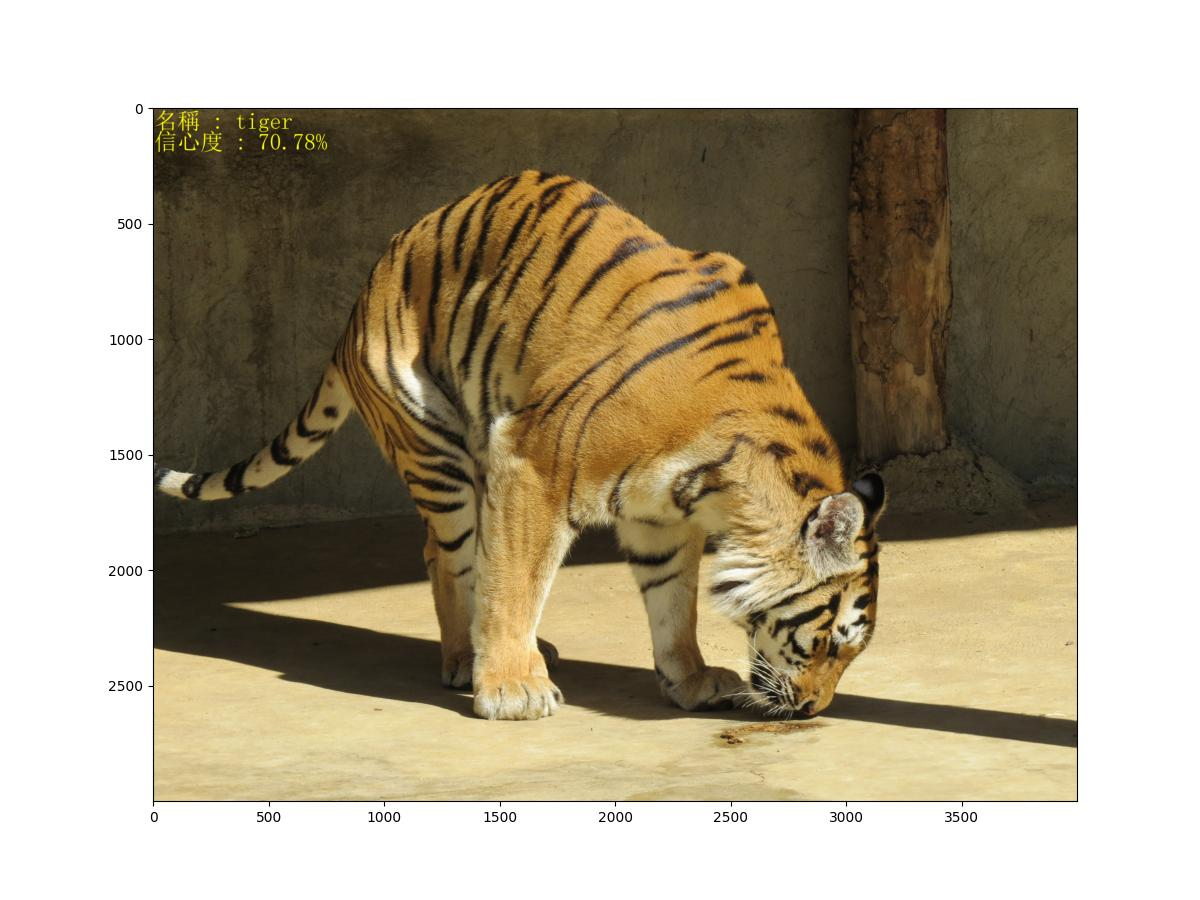# VGG19辨識

VGG16/19 是 ImageNet 每年舉辦的模型演算競賽(ILSVRC)中，於2014年獲得亞軍的模型。以前曾說明 VGG16 模型的運用，此篇改成 VGG19，其實用法差不多。VGG16/19 用來偵測圖片，將圖片進行分類，是由牛津大學計算機視覺組對 100 萬張圖片進行訓練，然後分成1000 個種類。

# 安裝套件

pip install tensorflow opencv-python Pillow matplotlib

# 簡易偵測

model=VGG19() 會建立模型，若是第一次執行，會到網路上下載模型，並儲存在 C:\Users\mahal\.keras\models 目錄中，所以會比較久。第二次執行，就不會重複下載，所以速度就快多了。

preprocess_input()稱為圖片預處理。預處理有三種方式，分別為 caffe, tf, troch。
VGG19採用 caffe方式，先將RGB 轉成 BGR格式，然後將所有點的B值減掉B的平均數，G及R亦同。如此就可以讓每個點的每個顏色值分佈在 0 的左右二邊。

predict() 偵測後，會傳回 1000 個分數，分別代表每一個種類的分數，分數愈高表示愈接近此分類。所以取得分數最高的那一個分類即可。

import os
os.environ['TF_CPP_MIN_LOG_LEVEL'] = '2'
import numpy as np
from PIL import Image, ImageFont, ImageDraw
from keras.applications import VGG19, vgg19
import cv2
from keras.applications.vgg19 import preprocess_input
import pylab as plt

model=VGG19(weights='imagenet', include_top=True)
x=cv2.resize(img, (224, 224), interpolation=cv2.INTER_LINEAR)
x=cv2.cvtColor(x, cv2.COLOR_BGR2RGB)

#VGG19規定在偵測(predict)時，必需傳入 #(1,224,224,3)的資料格式
x=np.expand_dims(x, axis=0)
#預處理圖片及偵測圖片
x=preprocess_input(x)
out=model.predict(x)

#decode_predictions轉成標籤及分數
results=vgg19.decode_predictions(out, top=3)
result=results
print('Predicted:', result)

#寫入文字並顯示圖片
pil = Image.fromarray(cv2.cvtColor(img, cv2.COLOR_BGR2RGB))
font = ImageFont.truetype('simsun.ttc', 100)
txt=f'名稱 : {result}\n信心度 : {result*100:.2f}%'
ImageDraw.Draw(pil).text((5, 5), txt, font=font, fill=(255,255,0))
plt.figure(figsize=(12,9))
plt.imshow(pil)
plt.show()